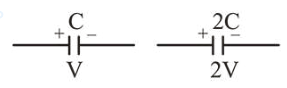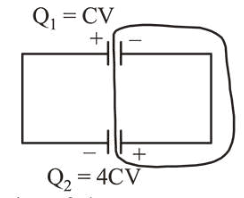# Two capacitors of capacitancesQuestion:

Two capacitors of capacitances $\mathrm{C}$ and $2 \mathrm{C}$ are charged to potential differences $\mathrm{V}$ and $2 \mathrm{~V}$, respectively. These are then connected in parallel in such a manner that the positive terminal of one is connected to the negative terminal of the other. The final energy of this configuration is :

1. (1) $\frac{25}{6} \mathrm{CV}^{2}$

2. (2) $\frac{3}{2} \mathrm{CV}^{2}$

3. (3) zero

4. (4) $\frac{9}{2} \mathrm{CV}^{2}$

Correct Option: , 2

Solution:

(b) When capacitors $C$ and $2 C$ capacitance are charged to $\mathrm{V}$ and $2 \mathrm{~V}$ respectively.$Q_{1}=C V \quad Q_{2}=2 C \times 2 V=4 C V$

When connected in parallelBy conservation of charge

$4 C V-C V=(C+2 C) V_{\text {common }}$

$V_{\text {common }}=\frac{3 C V}{3 C}=V$

Therefore final energy of this configuration,

$U_{f}=\left(\frac{1}{2} C V^{2}+\frac{1}{2} \times 2 C V^{2}\right)=\frac{3}{2} C V^{2}$